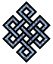fail2ban bad ip database: ip 200.44.50.155

| ip database | live view | stats | report | help | api key:

 ip: 200.44.50.155 hostname: 200-44-50-155.genericrev.cantv.net country:[VE] Venezuela first reported: 18.11.2018 22:18.35 GMT+0200 last reported: 07.03.2019 02:31.10 GMT+0200 time period: 108d 04h 12m 35s total reports: 12 reported by: 3 host(s) filter(s): ssh (11) ssh (1) tor exit node no badips.com db Lookupport scan of '200.44.50.155':

[-hide]
```# Nmap 6.40 scan initiated Sun Nov 18 22:19:01 2018 as: /usr/bin/nmap -sU -sS -O 200.44.50.155
Nmap scan report for 200-44-50-155.genericrev.cantv.net (200.44.50.155)
Host is up (0.16s latency).
Not shown: 999 open|filtered ports, 994 filtered ports
PORT    STATE  SERVICE
25/tcp  closed smtp
53/tcp  open   domain
80/tcp  closed http
110/tcp closed pop3
113/tcp closed ident
443/tcp closed https
53/udp  open   domain
No exact OS matches for host (If you know what OS is running on it, see http://nmap.org/submit/ ).
TCP/IP fingerprint:
OS:SCAN(V=6.40%E=4%D=11/18%OT=53%CT=25%CU=%PV=N%G=Y%TM=5BF1D786%P=x86_64-pc
OS:-linux-gnu)SEQ(SP=107%GCD=1%ISR=10D%TI=Z%TS=8)OPS(O1=M5B4ST11NW7%O2=M5B4
OS:ST11NW7%O3=M5B4NNT11NW7%O4=M5B4ST11NW7%O5=M5B4ST11NW7%O6=M5B4ST11)WIN(W1
OS:=16A0%W2=16A0%W3=16A0%W4=16A0%W5=16A0%W6=16A0)ECN(R=Y%DF=Y%TG=40%W=16D0%
OS:O=M5B4NNSNW7%CC=Y%Q=)T1(R=Y%DF=Y%TG=40%S=O%A=S+%F=AS%RD=0%Q=)T2(R=N)T3(R
OS:=N)T4(R=N)T5(R=Y%DF=Y%TG=40%W=0%S=Z%A=S+%F=AR%O=%RD=0%Q=)T6(R=N)T7(R=N)U
OS:1(R=N)IE(R=Y%DFI=N%TG=40%CD=S)

OS detection performed. Please report any incorrect results at http://nmap.org/submit/ .
# Nmap done at Sun Nov 18 22:20:06 2018 -- 1 IP address (1 host up) scanned in 65.07 seconds
```
```Σ = 65 | Δt = 0.0040378570556641s
```